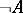# Decidable formula

(diff) ← Older revision | Latest revision (diff) | Newer revision → (diff)

(in a given system)

A formulaof a given formal system that is either provable in this system (that is, is a theorem) or refutable (that is, its negationis provable). If every closed formula of a given formal system is decidable, then such a system is said to be complete. (Note that it is impossible to require that all, and not just the closed, formulas be decidable in the system. Thus, the formula, whereruns over natural numbers, expresses neither a true nor a false proposition and therefore neither it nor its negation is a theorem of formal arithmetic.)

The name "decidable formula" comes from the fact that the truth or falsehood of the statement expressed by such a formula can be decided on the basis of the given system of axioms. By the Gödel incompleteness theorem there is an undecidable statement in any formal system of arithmetic, that is, a closed formula can be found that is not decidable in this system. In particular, the formula expressing the assertion that such a system is consistent turns out to be not decidable.

The term "decidable formula" should be distinguished from the term "decidable predicate" .